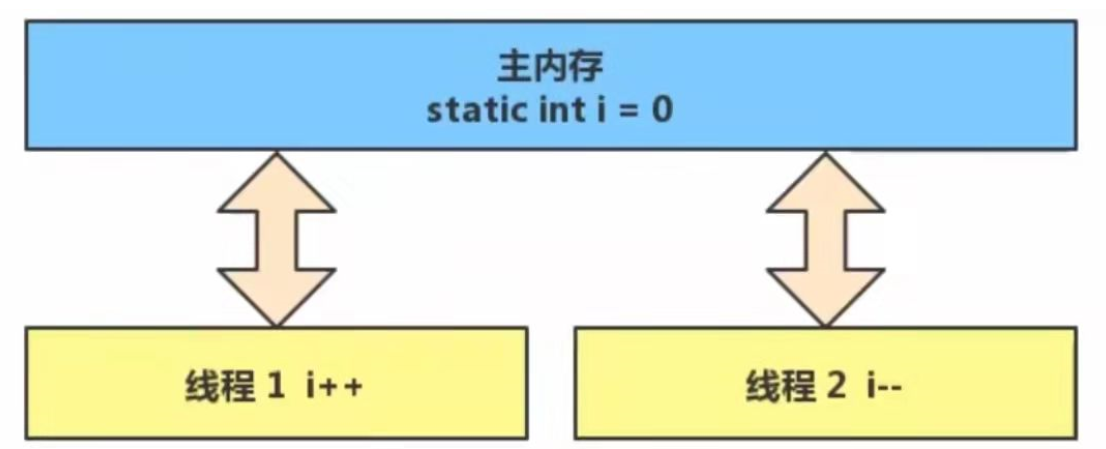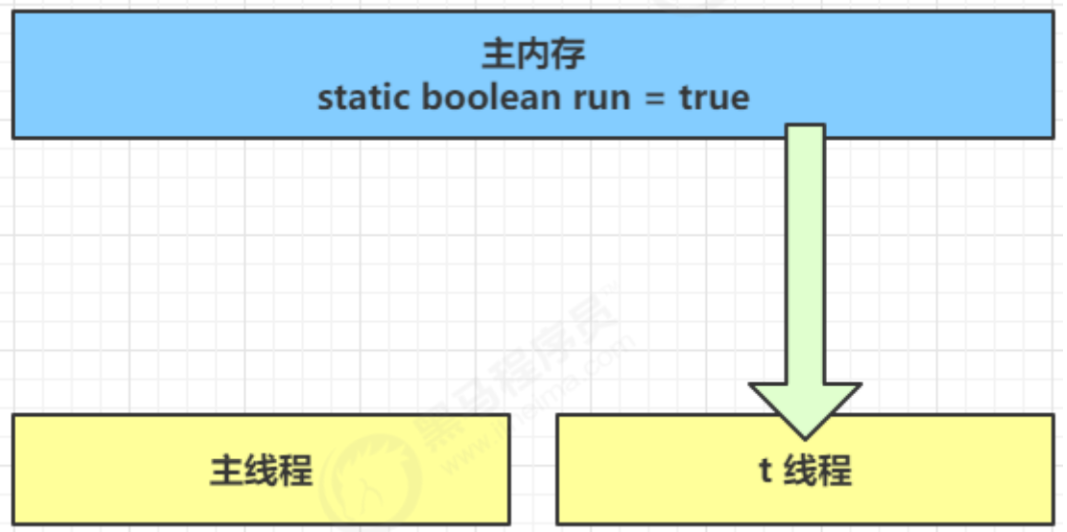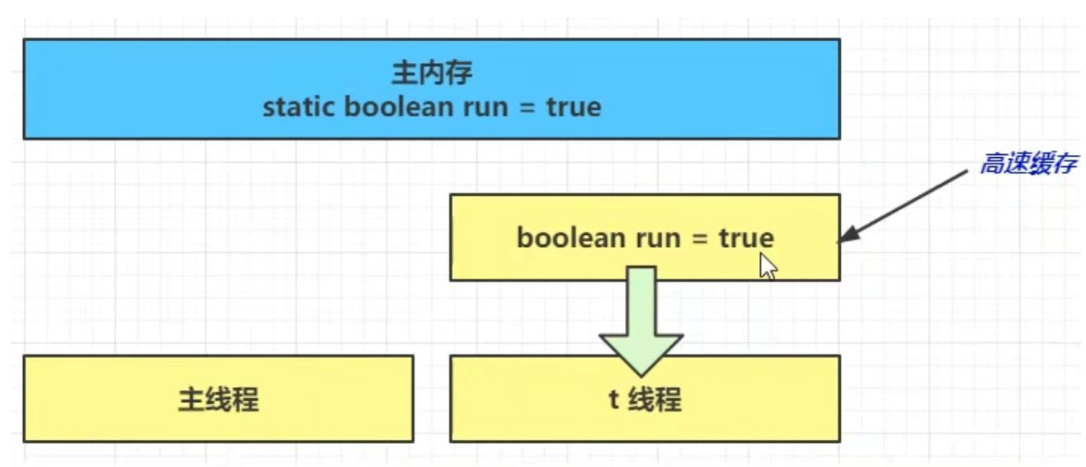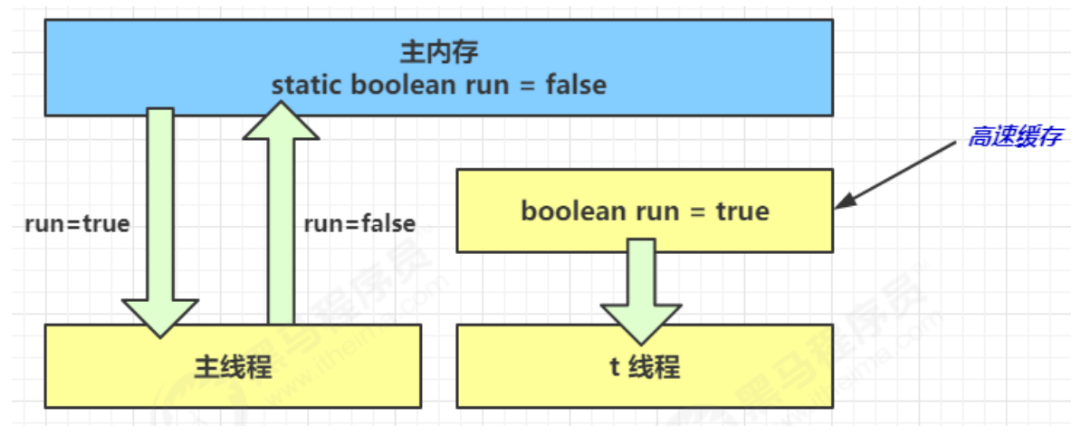WHCSRL 技术网

# （超详解）JVM-JMM内存模型

## JVM-JMM内存模型

• 很多人将【java 内存结构】与【java 内存模型】傻傻分不清，【java 内存模型】是 Java Memory Model（JMM）的意思。
• 简单的说，JMM 定义了一套在多线程读写共享数据时（成员变量、数组）时，对数据的可见性有序性、和原子性的规则和保障

## 1. 原子性

### 1-1 问题解析

``````public class Demo1 {
static int i = 0;

public static void main(String[] args) throws InterruptedException {

Thread t1 = new Thread(() -> {
for (int j = 0; j < 50000; j++) {
i++;
}
});
Thread t2 = new Thread(() -> {
for (int j = 0; j < 50000; j++) {
i--;
}
});
t1.start();
t2.start();
t1.join();
t2.join();
System.out.println(i);

}
}
```1234567891011121314151617181920212223```

``````getstatic i // 获取静态变量i的值
iconst_1 // 准备常量1
putstatic i // 将修改后的值存入静态变量i
```1234```

``````getstatic i // 获取静态变量i的值
iconst_1 // 准备常量1
isub // 减法
putstatic i // 将修改后的值存入静态变量i
```1234`````````// 假设i的初始值为0
getstatic i // 线程1-获取静态变量i的值 线程内i=0
iconst_1 // 线程1-准备常量1
iadd // 线程1-自增 线程内i=1
putstatic i // 线程1-将修改后的值存入静态变量i 静态变量i=1
getstatic i // 线程1-获取静态变量i的值 线程内i=1
iconst_1 // 线程1-准备常量1
isub // 线程1-自减 线程内i=0
putstatic i // 线程1-将修改后的值存入静态变量i 静态变量i=0
```123456789```

``````// 假设i的初始值为0
getstatic i // 线程1-获取静态变量i的值 线程内i=0
getstatic i // 线程2-获取静态变量i的值 线程内i=0
iconst_1 // 线程1-准备常量1
iadd // 线程1-自增 线程内i=1
putstatic i // 线程1-将修改后的值存入静态变量i 静态变量i=1
iconst_1 // 线程2-准备常量1
isub // 线程2-自减 线程内i=-1
putstatic i // 线程2-将修改后的值存入静态变量i 静态变量i=-1
```123456789```

``````// 假设i的初始值为0
getstatic i // 线程1-获取静态变量i的值 线程内i=0
getstatic i // 线程2-获取静态变量i的值 线程内i=0
iconst_1 // 线程1-准备常量1
iadd // 线程1-自增 线程内i=1
iconst_1 // 线程2-准备常量1
isub // 线程2-自减 线程内i=-1
putstatic i // 线程2-将修改后的值存入静态变量i 静态变量i=-1
putstatic i // 线程1-将修改后的值存入静态变量i 静态变量i=1
```123456789```

### 1-2 解决方法

#### （1）synchronized（同步关键字）

``````synchronized( 对象 ) {
要作为原子操作代码
}
```123```

`synchronized` 解决并发问题：

``````public class Demo1 {
static int i = 0;
static Object obj = new Object();

public static void main(String[] args) throws InterruptedException {

Thread t1 = new Thread(() -> {
for (int j = 0; j < 50000; j++) {
synchronized (obj) {
i++;
}

}
});
Thread t2 = new Thread(() -> {
for (int j = 0; j < 50000; j++) {
synchronized (obj) {
i--;
}
}
});
t1.start();
t2.start();
t1.join();
t2.join();
System.out.println(i);//输出为0
}
}
```12345678910111213141516171819202122232425262728```

## 2.可见性

### 2-1 退不出的循环

``````static boolean run = true;
public static void main(String[] args) throws InterruptedException {
while(run){
// ....
}
});
t.start();
run = false; // 线程t不会如预想的停下来
}
```1234567891011```

1. 初始状态， t 线程刚开始从主内存读取了 run 的值到工作内存。2. 因为 t 线程要频繁从主内存中读取 run 的值，JIT 编译器会将 run 的值缓存至自己工作内存中的高速缓存中，减少对主存中 run 的访问，提高效率1. 1 秒之后，main 线程修改了 run 的值，并同步至主存，而 t 是从自己工作内存中的高速缓存中读取这个变量的值，结果永远是旧值### 2-2 解决办法

#### （1）volatile（易变关键字）

``````public class Demo1 {
volatile static boolean run = true;

public static void main(String[] args) throws InterruptedException {
Thread t = new Thread(() -> {
while (run) {
// ....
}
});
t.start();
run = false; // 线程t不会如预想的停下来
}

}
```123456789101112131415```

`synchronized` 语句块既可以保证代码块的原子性，也同时保证代码块内变量的可见性。但 缺点是`synchronized`是属于重量级操作，性能相对更低

``````public void println(int x) {
synchronized (this) {
print(x);
newLine();
}
}
```123456```

## 3.有序性

### 3-1 诡异的结果

``````int num = 0;
boolean ready = false;
// 线程1 执行此方法
public void actor1(I_Result r) {
r.r1 = num + num;
} else {
r.r1 = 1;
}
}
// 线程2 执行此方法
public void actor2(I_Result r) {
num = 2;
}

```12345678910111213141516```

### 3-2 解决方法

volatile 修饰的变量，可以禁用指令重排

``````@JCStressTest
@Outcome(id = {"1", "4"}, expect = Expect.ACCEPTABLE, desc = "ok")
@Outcome(id = "0", expect = Expect.ACCEPTABLE_INTERESTING, desc = "!!!!")
@State
public class ConcurrencyTest {
int num = 0;
volatile boolean ready = false;//可以禁用指令重排
@Actor
public void actor1(I_Result r) {
r.r1 = num + num;
} else {
r.r1 = 1;
}
}
@Actor
public void actor2(I_Result r) {
num = 2;
}
}
```123456789101112131415161718192021```

### 3-3 有序性理解

``````static int i;
static int j;
// 在某个线程内执行如下赋值操作
i = ...; // 较为耗时的操作
j = ...;
```12345```

``````i = ...; // 较为耗时的操作
j = ...;
```12```

``````j = ...;
i = ...; // 较为耗时的操作
```12```

``````public class Singleton {
private Singleton() {
}

private static Singleton INSTANCE = null;

public static Singleton getInstance() {
//实例没创建，才会进入内部的 synchronized 代码块
if (INSTANCE == null) {
//可能第一个线程在synchronized 代码块还没创建完对象时，第二个线程已经到了这一步，所以里面还需要加上判断
synchronized (Singleton.class) {
//也许有其他线程已经创建实例，所以再判断一次
if (INSTANCE == null) {
INSTANCE = new Singleton();
}
}
}
return INSTANCE;
}
}
```1234567891011121314151617181920```

• 懒惰实例化
• 首次使用 getInstance() 才使用 synchronized 加锁，后续使用时无需加锁

`INSTANCE = new Singleton()` 对应的字节码为：

``````0: new #2 // class cn/itcast/jvm/t4/Singleton
3: dup
4: invokespecial #3 // Method "<init>":()V
7: putstatic #4 // Field INSTANCE:Lcn/itcast/jvm/t4/Singleton;
```1234```

``````时间1 t1 线程执行到 INSTANCE = new Singleton();

```123456```

### 3-4 happens-before

happens-before 规定了哪些写操作对其它线程的读操作可见，它是可见性有序性的一套规则总结，抛开以下 happens-before 规则，JMM 并不能保证一个线程对共享变量的写，对于其它线程对该共享变量的读可见

• 线程解锁 m 之前对变量的写，对于接下来对 m 加锁的其它线程对该变量的读可见

``````static int x;
static Object m = new Object();
synchronized(m) {
x = 10;
}
},"t1").start();
synchronized(m) {
System.out.println(x);
}
},"t2").start()
```123456789101112```
• 线程对 volatile 变量的写，对接下来其它线程对该变量的读可见

``````volatile static int x;
x = 10;
},"t1").start();
System.out.println(x);
},"t2").start();
```1234567```
• 线程 start 前对变量的写，对该线程开始后对该变量的读可见

``````static int x;
x = 10;
System.out.println(x);
},"t2").start();
```12345```
• 线程结束前对变量的写，对其它线程得知它结束后的读可见（比如其它线程调用 `t1.isAlive()``t1.join()`等待它结束）

``````static int x;
x = 10;
},"t1");
t1.start();
t1.join();
System.out.println(x);
```1234567```
• 线程 t1 打断 t2（interrupt）前对变量的写，对于其他线程得知 t2 被打断后对变量的读可见（通 过`t2.interrupted``t2.isInterrupted`

``````static int x;
public static void main(String[] args) {
while(true) {
System.out.println(x);//0
break;
}
}
},"t2");
t2.start();
try {
} catch (InterruptedException e) {
e.printStackTrace();
}
x = 10;
t2.interrupt();
},"t1").start();
while(!t2.isInterrupted()) {
}
System.out.println(x);//0
}
```12345678910111213141516171819202122232425```
• 对变量默认值（0，false，null）的写，对其它线程对该变量的读可见

• 具有传递性，如果 `x hb-> y` 并且 `y hb-> z` 那么有 `x hb-> z`

## 4.CAS与原子类

### 4-1 CAS

CAS`Compare and Swap` ，它体现的一种乐观锁的思想

``````// 需要不断尝试
while(true) {
int 旧值 = 共享变量 ; // 比如拿到了当前值 0
int 结果 = 旧值 + 1; // 在旧值 0 的基础上增加 1 ，正确结果是 1
/*
这时候如果别的线程把共享变量改成了 5，本线程的正确结果 1 就作废了，这时候
compareAndSwap 返回 false，重新尝试，直到：
compareAndSwap 返回 true，表示我本线程做修改的同时，别的线程没有干扰
*/
if( compareAndSwap ( 旧值, 结果 )) {
// 成功，退出循环
}
//不一样，继续循环尝试
}
```1234567891011121314```

• 因为没有使用 `synchronized`，所以线程不会陷入阻塞，这是效率提升的因素之一
• 但如果竞争激烈，可以想到重试必然频繁发生，反而效率会受影响

CAS 底层依赖于一个 `Unsafe` 类来直接调用操作系统底层的 CAS 指令，下面是直接使用 Unsafe 对象进行线程安全保护的一个例子：

``````public class TestCAS {
public static void main(String[] args) throws InterruptedException {
DataContainer dc = new DataContainer();
int count = 5;
Thread t = new Thread(() -> {
for (int i = 0; i < count; i++) {
dc.increase();
}
});
t.start();
t.join();
System.out.println(dc.getData());
}
}

class DataContainer {
private volatile int data;
static final Unsafe unsafe;
static final long DATA_OFFSET;

static {
try {
// Unsafe 对象不能直接调用，只能通过反射获得
Field theUnsafe = Unsafe.class.getDeclaredField("theUnsafe");
theUnsafe.setAccessible(true);
unsafe = (Unsafe) theUnsafe.get(null);
} catch (NoSuchFieldException | IllegalAccessException e) {
throw new Error(e);
}
try {
// data 属性在 DataContainer 对象中的偏移量，用于 Unsafe 直接访问该属性
DATA_OFFSET =
unsafe.objectFieldOffset(DataContainer.class.getDeclaredField("data"));
} catch (NoSuchFieldException e) {
throw new Error(e);
}
}

public void increase() {
int oldValue;
while (true) {
// 获取共享变量旧值，可以在这一行加入断点，修改 data 调试来加深理解
oldValue = data;
// cas 尝试修改 data 为 旧值 + 1，如果期间旧值被别的线程改了，返回 false
if (unsafe.compareAndSwapInt(this, DATA_OFFSET, oldValue, oldValue +
1)) {
return;
}
}
}

public void decrease() {
int oldValue;
while (true) {
oldValue = data;
if (unsafe.compareAndSwapInt(this, DATA_OFFSET, oldValue, oldValue -
1)) {
return;
}
}
}

public int getData() {
return data;
}
}
```123456789101112131415161718192021222324252627282930313233343536373839404142434445464748495051525354555657585960616263646566```

### 4-2 乐观锁与悲观锁

• CAS 是基于乐观锁的思想：最乐观的估计，不怕别的线程来修改共享变量，就算改了也没关系，我吃亏点再重试呗。
• synchronized 是基于悲观锁的思想：最悲观的估计，得防着其它线程来修改共享变量，我上了锁你们都别想改，我改完了解开锁，你们才有机会

### 4-3 原子操作类

juc`（java.util.concurrent）`中提供了原子操作类，可以提供线程安全的操作，例如：`AtomicInteger``AtomicBoolean`等，它们底层就是采用 CAS 技术 + volatile 来实现的。 可以使用 AtomicInteger 改写之前的例子：

``````public class TestCAS {
//创建原子整数对象
private static AtomicInteger i = new AtomicInteger(0);

public static void main(String[] args) throws InterruptedException {
Thread t1 = new Thread(() -> {
for (int j = 0; j < 5000; j++) {
i.getAndIncrement(); //获取并且自增 i++
}
});
Thread t2 = new Thread(() -> {
for (int j = 0; j < 5000; j++) {
i.getAndDecrement(); //获取并且自减 i--
}
});
t1.start();
t2.start();
t1.join();
t2.join();
System.out.println(i);//0
}
}
```12345678910111213141516171819202122```

## 5.synchronized 优化

Java HotSpot 虚拟机中，每个对象都有对象头（包括 `class 指针``Mark Word`）。`Mark Word` 平时存储这个对象的哈希码 、 分代年龄 ，当加锁时，这些信息就根据情况被替换为标记位 、 线程锁记录指针 、 重量级锁指针 、 线程ID 等内容

### 5-1 轻量级锁

``````static Object obj = new Object();
public static void method1() {
synchronized( obj ) {
// 同步块 A
method2();
}
}
public static void method2() {
synchronized( obj ) {
// 同步块 B
}
}
```123456789101112```

### 5-4 偏向锁

• 撤销偏向需要将持锁线程升级为轻量级锁，这个过程中所有线程需要暂停（STW）
• 访问对象的 hashCode 也会撤销偏向锁
• 如果对象虽然被多个线程访问，但没有竞争，这时偏向了线程 T1 的对象仍有机会重新偏向 T2，重偏向会重置对象的 Thread ID
• 撤销偏向和重偏向都是批量进行的，以类为单位
• 如果撤销偏向到达某个阈值，整个类的所有对象都会变为不可偏向的
• 可以主动使用 `-XX:-UseBiasedLocking` 禁用偏向锁

### 5-5 其他优化

#### （2）减少锁的粒度

• `ConcurrentHashMap`
• `LongAdder` 分为 `base``cells` 两部分。没有并发争用的时候或者是 cells 数组正在初始化的时候，会使用 CAS 来累加到base，有并发争用，会初始化 cells 数组，数组有多少个 cell，就允许有多少线程并行修改，最后将数组中每个 cell 累加，再加上 base 就是最终的值
• `LinkedBlockingQueue` 入队和出队使用不同的锁，相对于`LinkedBlockingArray`只有一个锁效率要高

#### （3）锁粗化

``````new StringBuffer().append("a").append("b").append("c");
```1```

#### （4）锁消除

JVM 会进行代码的逃逸分析，例如某个加锁对象是方法内局部变量，不会被其它线程所访问到，这时候就会被即时编译器忽略掉所有同步操作。

#### （5）读写分离

• 可以主动使用 `-XX:-UseBiasedLocking` 禁用偏向锁